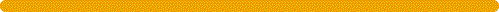#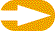Solar zenith angle

The solar zenith anglez is a function of time, day number and latitude. It can be calculated using the relation:in whichis the declination of the sun,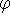the latitude (defined as positive in the northern hemisphere) and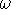the hour angle. The latter is a measure of the local time, i.e. it is defined as the angle through which the earth must turn to bring the meridian of the location of observation directly under the sun. The solar declination is a function of the day of the year and is independent of the location. It varies from 23o27' on June 21 to -23o27' on December 22.

At noon at any latitude cos() = 0 andz=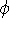-. At sunrise or sunset at any latitude except the North or South Poles cos(z ) = 0, and= Nd/2 = half-day length (expressed in rad, i.e., 2Ācorresponds to 24 h), andThe day length will be 12 hours if either tan() = 0 (the equator on all days) or tan() = 0 (the equinoxes at all latitudes except the Poles). The latitude of the polar night may be found by setting Nd = 0 in the above equation, leading to 90o-||=in the winter hemisphere.

At the Poles cos() = 0, sin() = 1, and cos(z ) = sin() or (in degrees): 90o -z =. Hence, at these points the elevation angle of the sun always equals the declination angle and during 6 months of daylight the sun simply circles around the horizon, never rising more than 23o27' above it.